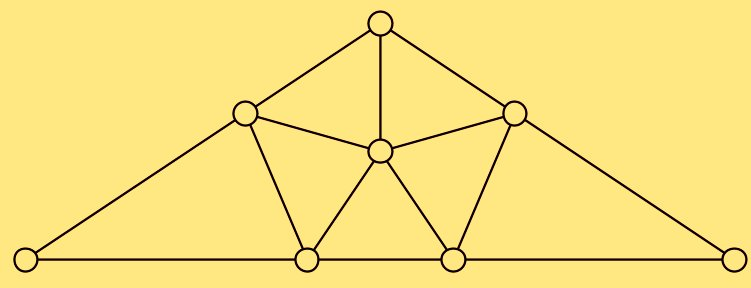# Acute triangulation

Assume that $S$ is a finite 2-dimensional simplicial complex equipped with a metric $d$ such that each triangle is isometric to a plane triangle (so $(S,d)$ is a polyhedral space).

Is it possible to subdivide the triangulation of $S$ so that it will have acute triangles only?

• It is well known that any triangle admits a triangulation with only acute triangels; one can see the proof in the following picture. But I do not see a way to fit such triangulations together.• Such a triangulation would be useful in the proof of Zalgaller's theorem: any n-dimensional polyhedral space admits a length-preserving piecewise linear map to $\mathbb R^n$. Well, it would help only if $n=2$, for larger $n$, we should say that a simplex is acute if it contains its circumcenter.

• Related though you are probably aware of it: It is shown in [Kopczynski, Pak, Przytycki, Acute triangulations of polyhedra and $\mathbb{R}^n$][mimuw.edu.pl/~pprzytyc/combinatorica.pdf] that the 4-cube and $\mathbb{R}^n$ for $n\ge 5$ do not admit acute triangulations. Where acute means in their sense that all dihedral angles are smaller than 90°. – HenrikRüping May 15 '12 at 14:11